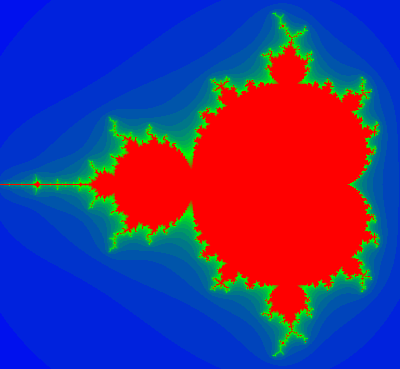In “Geometric Algebra for Computer Science” is a fractal problem based on a vectorization of the Mandelbrot equation, which allows for generalization to $$N$$ dimensions.

I finally got around to trying the 3D variation of this problem.  Recall that the Mandlebrot set is a visualization of iteration of the following complex number equation:
\begin{equation}
z \rightarrow z^2 + c,
\end{equation}
where the idea is that $$z$$ starts as the constant $$c$$, and if this sequence converges to zero, then the point $$c$$ is in the set.

The idea in the problem is that this equation can be cast as a vector equation, instead of a complex number equation. All we have to do is set $$z = \Be_1 \Bx$$, where $$\Be_1$$ is the x-axis unit vector, and $$\Bx$$ is an $$\mathbb{R}^2$$ vector. Expanding in coordinates, with $$\Bx = \Be_1 x + \Be_2 y$$, we have
\begin{equation}
z
= \Be_1 \lr{ \Be_1 x + \Be_2 y }
= x + \Be_1 \Be_2 y,
\end{equation}
but since the bivector $$\Be_1 \Be_2$$ squares to $$-1$$, we can represent complex numbers as even grade multivectors. Making the same substitution in the Mandlebrot equation, we have
\begin{equation}
\Be_1 \Bx \rightarrow \Be_1 \Bx \Be_1 \Bx + \Be_1 \Bc,
\end{equation}
or
\begin{equation}
\Bx \rightarrow \Bx \Be_1 \Bx + \Bc.
\end{equation}
Viola! This is a vector version of the Mandlebrot equation, and we can use it in 2 or 3 or N dimensions, as desired.  Observe that despite all the vector products above, the result is still a vector since $$\Bx \Be_1 \Bx$$ is the geometric algebra form of a reflection of $$\Bx$$ about the x-axis.

The problem with generalizing this from 2D is really one of visualization. How can we visualize a 3D Mandelbrot set? One idea I had was to use ray tracing, so that only the points on the surface from the desired viewpoint need be evaluated. I don’t think I’ve ever written a ray tracer, but I thought that there has got to be a quick and dirty way to do this.  Also, figuring out how to make a ray tracer interact with an irregular surface like this is probably non trivial!

What I did instead, was a brute force evaluation of all the points in the upper half plane in around the origin, one slice of the set at a time. Here’s the resultCode for the visualization can be found in github. I’ve used Pauli matrices to do the evaluation, which is actually pretty quick (but slower than plain std::complex< double> evaluation), and the C++ ImageMagick API to save individual png files for the slices. There are better coloring schemes for the Mandelbrot set, and if I scrounge up some more time, I may try one of those instead.